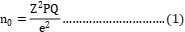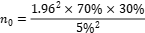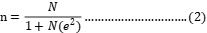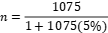Blog# HOW TO SELECT THE SAMPLE AND CALCULATE SAMPLE SIZE FOR SURVEY

Introduction

In most cases, such as when conducting a questionnaire survey, it would be impractical to research the entire population. Sampling is a technique that allows researchers to infer knowledge about a population from the findings of a subset of the population rather than trying to look at every single individual. Reducing the number of participants in a study lowers costs and workload, and it may make it easier to collect high-quality data, but this must be balanced against providing a large enough sample size with sufficient power to detect a true correlation.

Method of Selecting Sample

There are several types of sampling methods, which can be classified into two categories: probability sampling and non-probability sampling. You begin with a full sampling frame of all eligible individuals from which to pick your sample in probability (random) sampling. As a result, all qualifying individuals will have a better chance of being selected for the survey, and you will be able to generalize the findings of your research. Probability sampling methods take longer and cost more money than non-probability sampling methods. Simple random sampling, systematic sampling, stratified sampling, and clustered sampling are some examples.

Since you don’t start with a full sampling frame in non-probability (non-random) sampling, some people have no chance of being chosen. As a consequence, the effect of sampling error cannot be estimated, and there is a substantial risk of producing a non-representative sample with non-generalizable effects. Non-probability sampling methods, on the other hand, are less expensive and more compact, making them ideal for exploratory study and hypothesis generation. Convenience sampling, quota sampling, judgment (or purposeful) sampling, and snowball sampling are some examples.

How to Calculate Sample Size

Two formulas are commonly used in the calculation of Sample size.  This includes Cochran 1977, and Yamane 1966 formula. Both were used to illustrate an example using a Population of 1075 RespondentsOption One: Using Cochran (1963) the sample size can be determined using the formula:

Where,

= Sample Size (Units),

= Abscissa of the normal curve that cuts off an area α at the tails (1 – α equals the desired confidence level, e.g., 95%),

P = Estimated Proportion of an Attribute that is Present in the Population,

Q = 1-P = 70%, and

= Desired Level of Precision = 5%.

Hence,This will give a total sample size of 322 RespondentsOption Two: Using Yamane formula

The study used Yamane (1967) for estimating sample size:Where,

n = Sample Size (Units)

N= Sample Frame/Population size (Units)

e = Level of Precision (5%)

This will give a total sample size of 291 Respondents

References

Cochran, W. G. (1963). Sampling Techniques, 2nd Ed., New York: John Wiley and Sons, Inc.

Yamane, T. (1967). Statistics, An Introductory Analysis, 2nd ed., New York: Harper and Row.

Author

Daniel PETER
Ph.D. Agricultural Economics (in view)